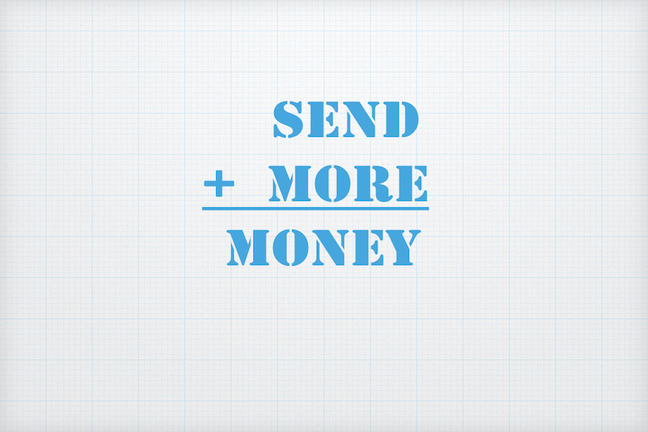1.12

Weizmann Institute of ScienceA famous cryptarithm

What are alphametics?

Alphametics are cryptarithms that spell out words. Given a mathematical expression, every digit in the expression is replaced by a letter. One of the most famous alphametics, spelling out ‘SEND MORE MONEY’ appears above. This alphametic was first published by Henry Dudeney, a British puzzlist, in 1924.

Five rules govern alphametics:

1. Identical digits are replaced by the same letter. Different digits are replaced by different letters.
2. After replacing all the letters with digits, the resulting arithmetic expression must be mathematically correct.
3. Numbers cannot start with 0. For example, the number 0900 is illegal.
4. Each problem must have exactly one solution, unless stated otherwise (unlike the “Hindu” problems where there might be no solution or multiple solutions).
5. The problems will be in base 10 unless otherwise specified. This means that the letters replace some or all of the 10 digits – 0, 1, 2, 3, 4, 5, 6, 7, 8, 9.